# High School Math : Cubes

## Example Questions

### Example Question #7 : How To Find The Volume Of A Cube

A building is in the shape of a perfect cube, with a side length of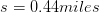. What is the building's volume, in cubic meters?

Not enough information to solve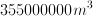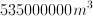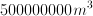Explanation:

In order to solve this question, we need to convert miles to meters via dimensional analysis and then solve for the volume of the building.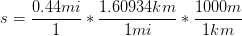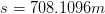Now, solve for volume.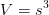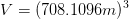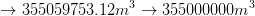### Example Question #8 : How To Find The Volume Of A Cube

This figure is a cube with one face having an area of 16 in2.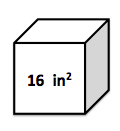What is the volume of the cube (in3)?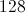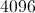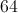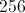Explanation:

The volume of a cube is one side cubed.  Because we know that one face has an area of 16 in2, then we know that one side must be the square root of 16 or 4.  Thus the volume is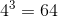.

### Example Question #9 : How To Find The Volume Of A Cube

A cube has a height of 4 feet.  What is the volume of the cube in feet?Explanation:

We only have the length of one edge, but that is sufficient for finding the volume.  The volume of a cube equals one edge multiplied by itself three times: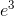### Example Question #10 : How To Find The Volume Of A Cube

A cube has a surface area of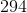units squared. What is its volume?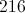units cubed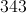units cubed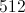units cubed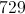units cubedunits cubed

Explanation:

Since a cube hassquare faces and the surface area of each face is given by multiplying the length of one side of the square face by itself, the equation for the surface area of a cube is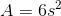, whereis the length of one side of one face (i.e., one edge of the cube). Find the length of one side/edge of the given cube by setting the given surface area equal to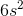: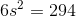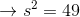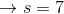units

The volume of a cube is, whereis the length of one of the cube's edges. Substituing the solution to the previous equation forin the volume equation gives the volume of the cube: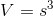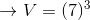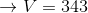units cubed

### Example Question #11 : How To Find The Volume Of A Cube

Find the volume of the following cube: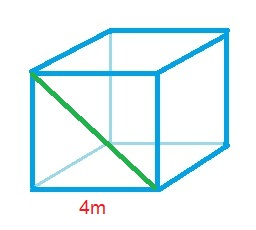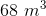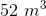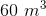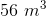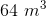Explanation:

The formula for the volume of a cube is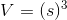,

whereis the side of the cube.

Plugging in our values, we get: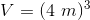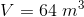### Example Question #12 : How To Find The Volume Of A Cube

What is the difference in volume between a sphere with radius x and a cube with a side of 2x? Let π = 3.14

8.00x3

6.73x3

3.82x3

4.18x3

5.28x3

3.82x3

Explanation:

Vcube = s3 = (2x)3 = 8x3

Vsphere = 4/3 πr3 = 4/3•3.14•x3 = 4.18x

### Example Question #13 : How To Find The Volume Of A Cube

The density of gold is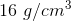and the density of glass is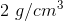.  You have a gold cube that is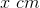in length on each side.  If you want to make a glass cube that is the same weight as the gold cube, how long must each side of the glass cube be?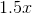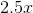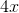Explanation:

Weight = Density * Volume

Volume of Gold Cube = side3= x3

Weight of Gold = 16 g/cm3 * x3

Weight of Glass = 3/cm3  * side3

Set the weight of the gold equal to the weight of the glass and solve for the side length:

16* x3 = 2  * side3

side3 = 16/2* x3 =  8 x3

Take the cube root of both sides:

side = 2x

### Example Question #1 : How To Find The Volume Of A Cube

A cube has edges that are three times as long as those of a smaller cube. The volume of the bigger cube is how many times larger than that of the smaller cube?Explanation:

If we letrepresent the length of an edge on the smaller cube, its volume is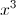.

The larger cube has edges three times as long, so the length can be represented as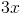. The volume is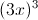, which is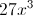.

The large cube's volume ofis 27 times as large as the small cube's volume of.

### Example Question #10 : How To Find The Volume Of A Cube

If a cube has its edges increased by a factor of 5, what is the ratio of the new volume to the old volume?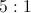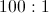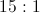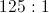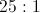Explanation:

A cubic volume is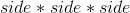. Let the original sides be 1, so that the original volume is 1. Then find the volume if the sides measure 5.  This new volume is 125.  Therefore, the ratio of new volume to old volume is 125: 1.

### Example Question #14 : How To Find The Volume Of A Cube

A cube has a side length ofmeters. What is the volume of the cube?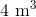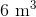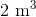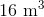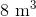Explanation:

The formula for the volume of a cube is: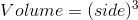Since the length of one side ismeters, the volume of the cube is: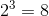meters cubed.